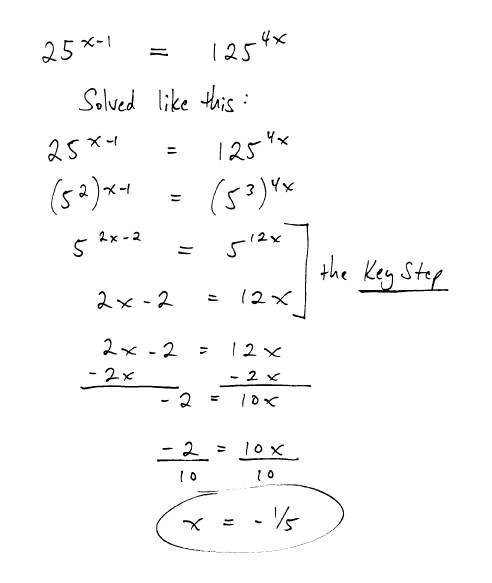## Kiss those Math Headaches GOODBYE!### Rubik’s Slide: play your way to geometric knowledge

A toy that educates … could it be a dream?

I recently found something that fits that category, educating students in concepts of GEOMETRY.

It’s called the Rubik’s Slide, created by Techno Source. I bought this Rubik’s Slide a few months ago because I needed another puzzle to keep my tutoring clients entertained while I grade their work, which I often do at the start of sessions.

### Finding domain and range — with color!

Have you ever noticed that a lot of students struggle with the idea of domain and range? This concept, taught mostly in Algebra 2,  often confuses students to the point where they cannot even identify the domain and range of a simple, continuous function.

I don’t really understand why students struggle with this concept, but I recently found a way of showing the idea that makes it considerably easier — using color to mark up a function.

Here’s an example of a problem where students need to figure out the domain and range by looking at a graph, like this:What I have students do is use two colors to sort of “box in” the function. With one color, green in this case, students mark the left bound and right bound of the function by drawing vertical lines. And with another color, red, students mark the lower bound and upper bound by  drawing horizontal lines. I have students write in the phrases:  left bound, right bound, lower bound, and upper bound, like this:Finally I ask students to figure out the domain and range by writing three-part inequalities for x and y, respectively, like this:I’ve used this approach with a number of students, and so far no one has been unable to find the domain and range when using it. So it appears to be a winner. Try it yourself, either as you teach a concept, or as you re-teach it to those who are struggling.

### Using Color to Show Perimeter

Many things look better in color, right?

So why should that be any different  in math?

I’ve found that taking a “colorful approach” to math not only makes mathematical objects look more interesting and pleasurable, it can also make mathematical concepts more clear.

Here’s an example from something I did today — I used color to show a shortcut for finding the perimeter of rectangular-ish objects.

I was tutoring a boy who had to find the perimeter of this figure:

This student did not see that there is a short-cut that could help him find the perimeter. I wanted to make this clear, so I reached for my color pencils and colorized both the left vertical segment and the two right vertical segments. My goal was to help the student see that the sum of the two right vertical segments equals the long left vertical segment.The student realized this after I colorized it. Then I used a different color, red, to show that the sum of the two horizontal segments on top equals the longer horizontal bottom segment, like this:At this point I felt that the student was ready to see the math that relates to the whole figure, so I wrote the math, using color to relate the numbers to the colors of the sides of the figure, like this:At this point the student was able to see the shortcut in this kind of problem, which together we wrote as follows:This is a fairly basic example of how color can, quickly and effectively, illustrate math concepts. Feel free to share examples of how you use color in your math lessons. I’m curious to learn (and share) a variety of ways, for I see that color has great potential.

### How to Divide Fractions: from annoying to FUN!

O.K., I’m ready to share my amazing approach to dividing a fraction by another fraction. Well, maybe not breathtaking … like Andrew Wiles’ proof of Fermat’s Last Theorem … but at least interesting. And best of all, fun and student-friendly!

Last week I asked if anyone had any tricks up their sleeves that make it easier for students to divide fractions. And I said that I would share a trick after I heard from you.

I got a nice response from Michelle, who said that she has used the mnemonic “KFC” (like the fried chicken), which in her class stands for Keep-Change-Flip. The idea being that you KEEP the first fraction, and next you CHANGE the sign from multiplication to division. Finally you FLIP the second fraction, the fraction on the right. We have similar mnemonic where I live, which goes by the phrase: Copy-Dot-Flip, with the “dot” meaning the dot of multiplication.

But what I want to share with you is a completely different approach to dividing one fraction by another, an approach that saves time, and makes it both easier and more fun — in my humble opinion — than the standard approach.

The approach I’m going to show you works for any complex fraction situation you might encounter, such as these: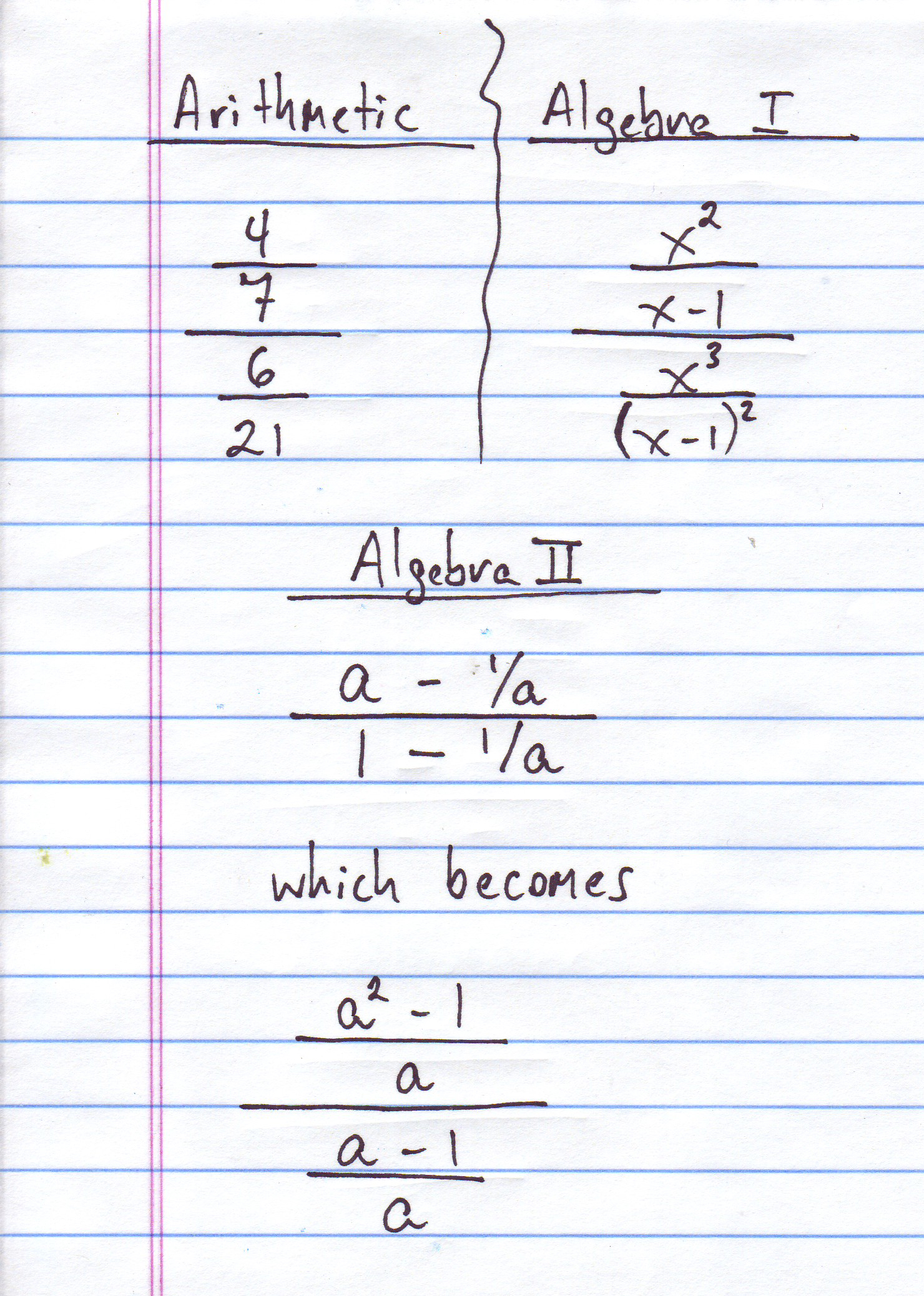For this blog post, I’m going to limit my chat to complex fractions of the arithmetic type, meaning those with numbers only, and no variables. And if it seems important, I’ll do another post later on using this very same process for algebraic fractions.

So what is this amazing approach, anyway? Well, it’s based on something I discovered on day when I was just messing around with fractions divided by fractions. I realized that after you do the KFC or the Copy-Dot-Flip, what you get — in general — is actually something really easy to grasp, as this next image will show you, along with a Quick Proof:If you take a moment to think about it, the terms in the numerator of the result — terms a and d — have something in common; they were on the outside of the original complex fraction, so I call these terms the “outers.” In the same way, the terms in the denominator of the result — terms b and c — were both on the inside of the complex fraction, so I call them the “inners.”

So when you divide fractions in this vertical format, the answer is simply the outers, multiplying each other divided by the inners, multiplying each other.

I find that students find this easy to remember and a cinch to do. This next sheet summarizes the idea, and also provides a fun way of remembering the concept, thinking about the stack of terms as a fraction “sandwich.”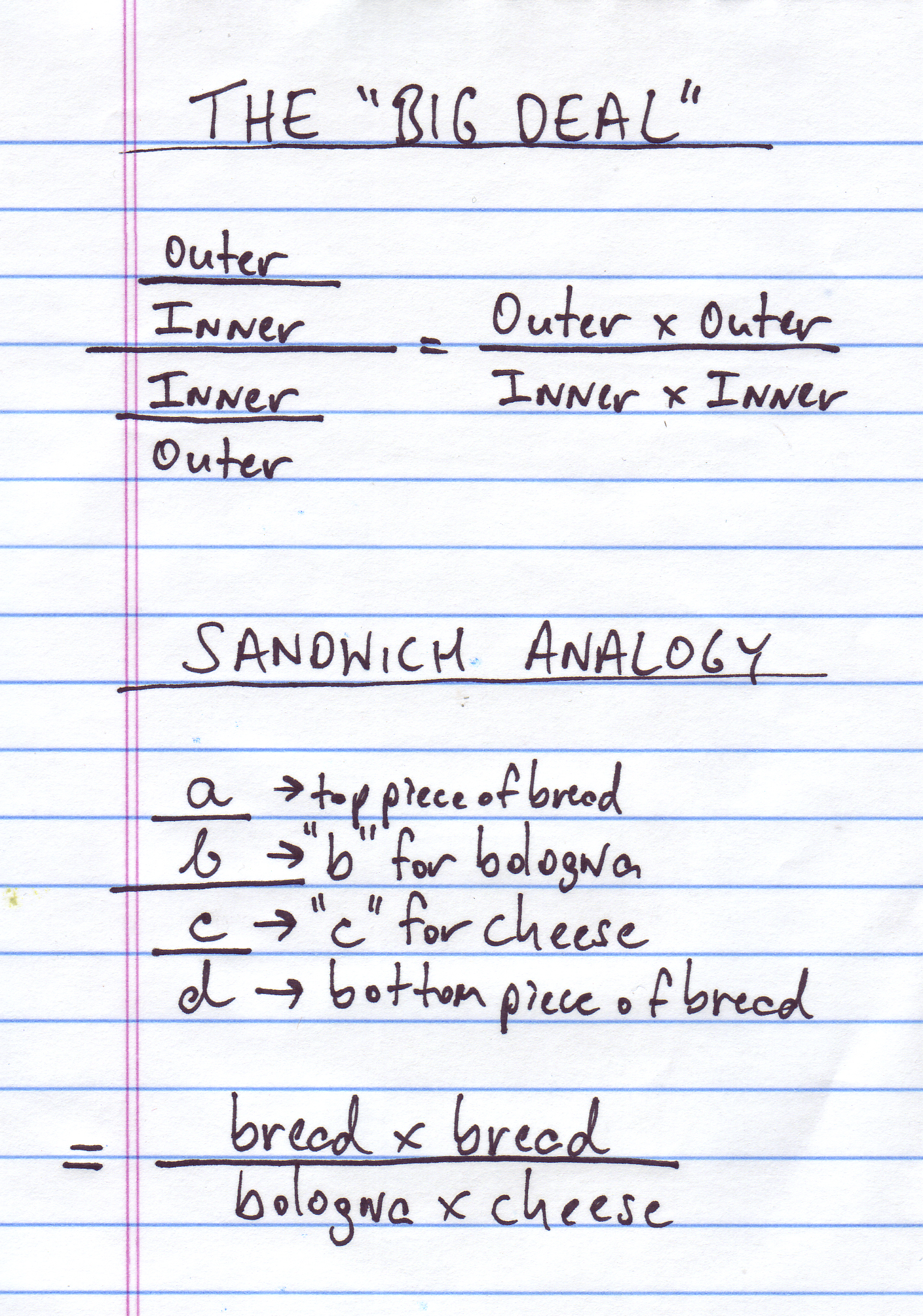So, to put this in words, the four-level complex fraction that you start out with can be thought of as a sandwich, with two pieces of bread at top and bottom, and slices of bologna and cheese in the middle.

The main point is that to simplify the fraction sandwich, all you need to do is put the two slices of bread together in the numerator and multiply them, And then put the bologna and cheese together in the denominator, and multiply them.

Using this idea it becomes a lot easier to simplify these complex fractions. Here’s an image that shows how it is done, and how this approach saves time over the way we were taught to do it, using reciprocals.And there’s more good news. This new way of looking at complex fractions also gives students a cool, new way to simplify the fractions before they get the answer. And when you do simplify fully, the answer you get will be a fraction that’s already completely reduced, so you won’t have to stress about that part.

The next two pages show you this fun and easy new way to simplify: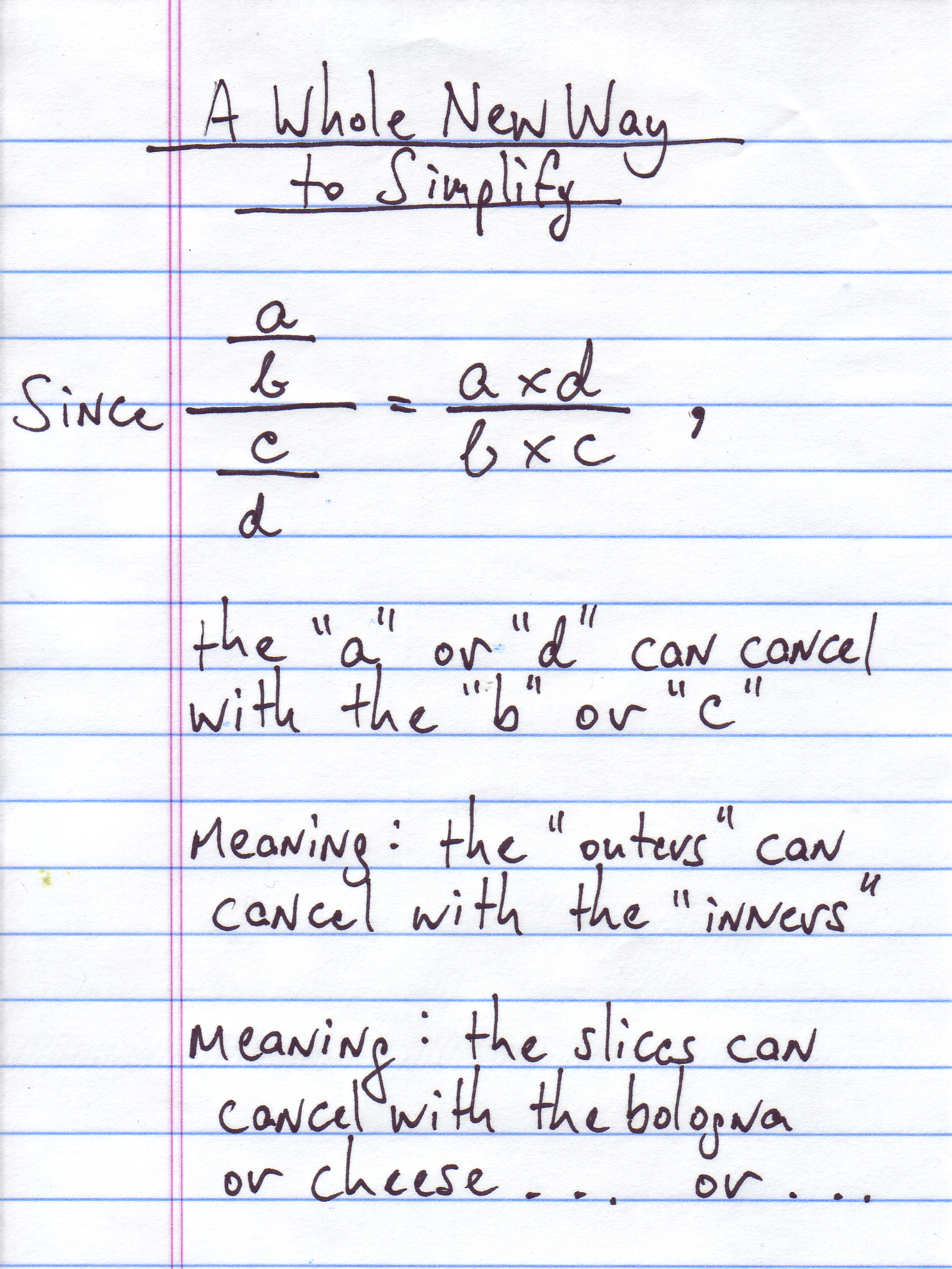or, or what? …  Here’s what …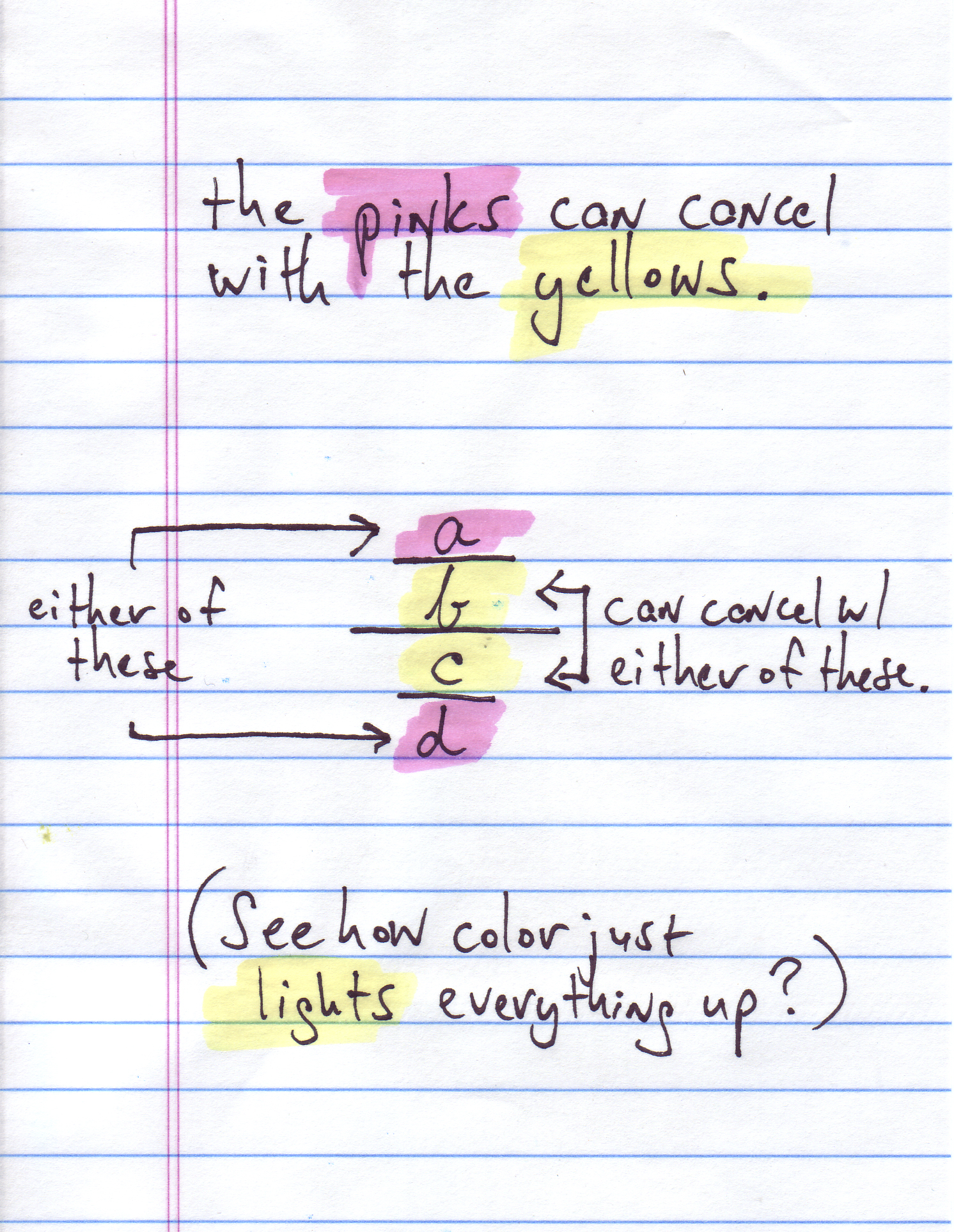So now you might like to see the whole process from start to finish, so you can decide for yourself if this technique is for you. Well that’s exactly what we’re showing next. As you can see I consistently highlight the outers with pink, the inners with yellow.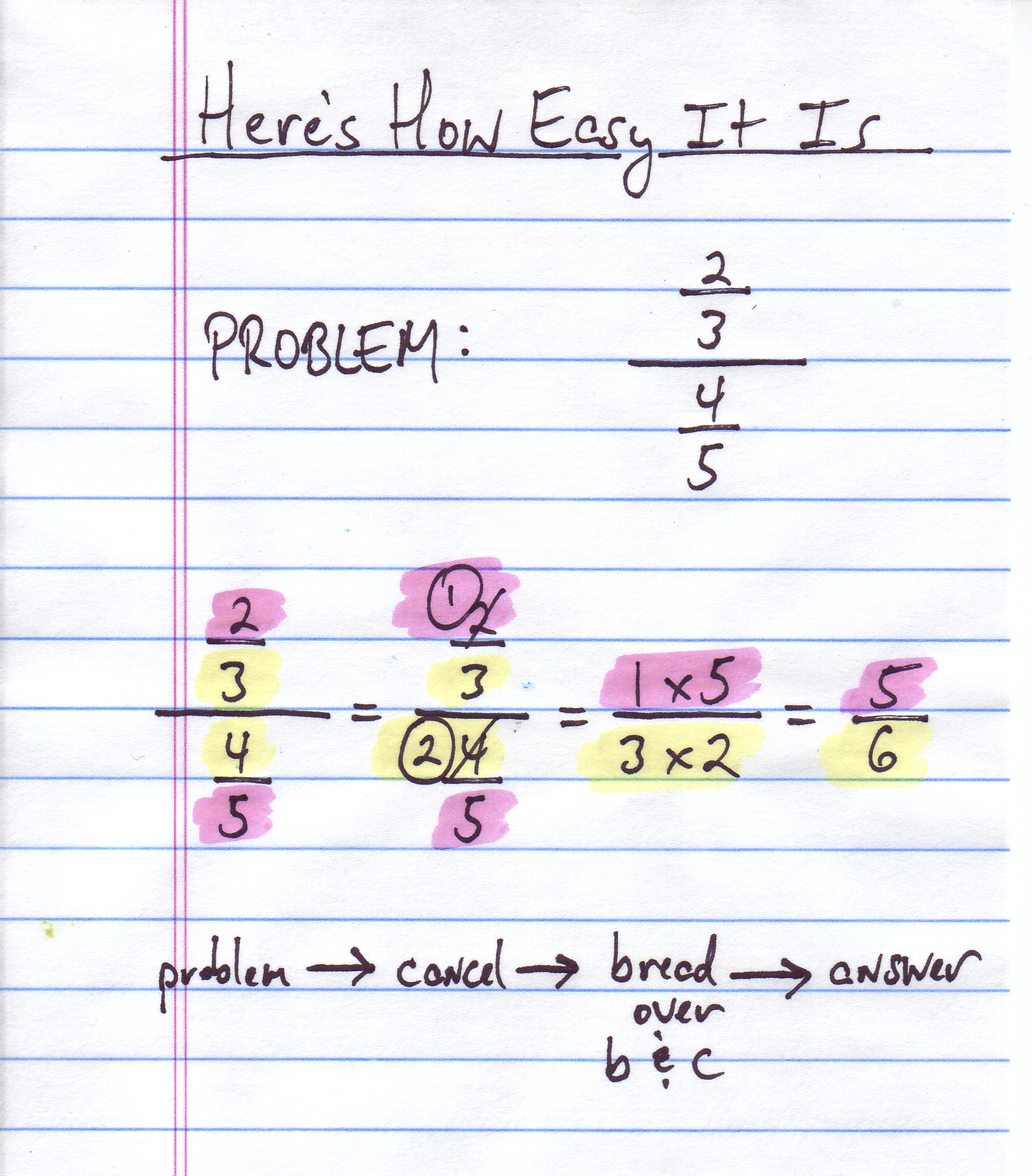And finally, a “harder” problem, you might say. But check it out. Is it really any harder than the one we’ve just done? You decide.In my next blog I’ll give you a few problems like these, so you can get used to this trick, and start shaving precious seconds and nano-seconds off the time it take you to do your homework, so you spend more time doing all of those things that you want to do more:  texting, watching You-Tube, taking hikes, skating (roller and ice), etc. etc. , etc. You know better than me.

Happy Teaching and Learning!

—  Josh

Josh Rappaport is the author of five books on math, including the Parents Choice-award winning Algebra Survival Guide. If you like how Josh explains these problems, you’ll certainly  like the Algebra Survival Guide and companion Workbook, both of which are available on Amazon.com  Just click the links in the sidebar for more information!### Algebra mistakes due to Negative Signs? Use COLOR to EXPLAIN.

Have you ever noticed how much trouble students have figuring out the difference between these two kinds of expressions: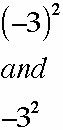The key, I’ve found, is to use the Order of Operations to decode the meaning of each expression.

Ask your students to think through how many Order of Operation steps there are in each expression.

If they’re on the right track, they’ll see that the top expression has just one operation:  working out the exponent by multiplying (– 3) by itself twice. This looks like this:As for the bottom expression, this is more difficult because of that “loose” negative sign, a negative sign that is not enclosed inside parentheses. Just like a “loose canon” can be a danger in the military, a “loose” negative sign can spell trouble on the algebra playing-field. Students will make all kinds of weird mistakes in trying to figure out what to do with this little varmint.

But if you tell students to view that “loose” negative sign as meaning (– 1) times the expression that follows it, they can use the Order of Operations again to do the right thing first.

Specifically, they notice that there are two operations to be performed: multiplication and exponents, and they remember that they do exponents before multiplication, following the Order of Operations.

Following these steps, the simplification looks like this:And while this is fine and correct, notice that you can “kick it up a notch” by using color to separate out the two operations. I use blue for decoding the negative sign and working it out; I use red for the 3-squared term. Check it out: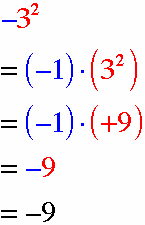I’ve yet to meet a student who cannot follow this procedure, when color is used.

Try it out and see what kind of results you get.

### Color Your Way to Integer Success

We all know that one of the trickiest subject for many students is the subject of combining integers.

I’ve hit on a new way to help students with this topic, a way that involves using color.

Using different colors helps students relate similar concepts and separate different concepts. Color works faster than underlining or drawing rings around numbers, it’s more attractive, and it makes a student’s page fun to look at, too.

Here’s just one example of how using color can help students make sense of those oft-bewildering positive and negative numbers.

Take a problem like:   + 3 – 7 + 6 – 9

Many students get confused by a problem like this because they don’t have the least idea of what to do first.

But when you use color, tell students that the first step is an easy step:  just re-write the problem, making the positive numbers red, and the negative numbers blue, like this:

The next step is equally easy … group the positives on the left and the negatives on the right. I use my handy-dandy “double-slash” divider to show the separation, like this:After that, use the rule for combining integers with the same sign (here you can say “with the same color!”), like this:

At this point you depart from color, as you combine the integers with different signs, and you get the answer, like this:

All together, it looks like this:

I have found this approach extremely helpful for those students — and you know the ones I’m talking about — who just struggle endlessly with these rules.

By the way, if you’d like to see the chapter of my Algebra Survival Guide, which explains the “Same-Sign” Rule and the “Mixed-Sign” Rule, just go to this page and scroll down about half-way down till you come to the link for downloading chapters:

http://singingturtle.com/pages/PARENTS3.html

Please try this out yourself and feel free to let me know how it goes. I’m always open to feedback.

### How to Use Color to Explain Algebra Concepts

Lately I’ve been having great success using COLOR to explain algebraic concepts, as strange as that may seem.

I’ve found that is that adding color to equations helps students see important relationships among the terms, for it lets them visually group related terms.

This is impossible to explain without an example, right? So here’s one to show you how it’s done.

GOAL: Give students a way of understanding the following exponent rule, usually learned in Algebra II:In explaining this rule, I first show students a common sense but critically important idea:

GIVEN: Two terms are equal to each other, and each term has two parts.

CONCLUSION: IF two of the parts are equal (blue), you can CONCLUDE that the other parts are also equal (pink).

Here’s a visual WITH COLOR that gets this idea across: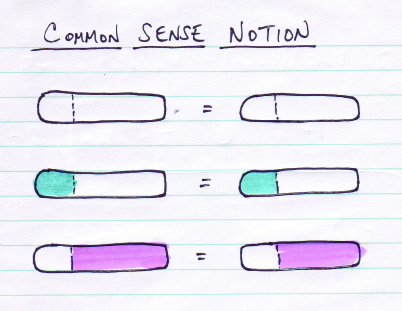Next I help students recall an early math concept that uses this principle. In this case, the following example gives them a nice way of seeing this concept with color:The conclusion, of course, is that x + 4 = 9, meaning that x = 5.

Notice that I consistently use the same colors to indicate the same ideas: blue, to show the parts that we know are equal; pink, to indicate the parts that we conclude are equal.

Finally I use this same color scheme to show the logic behind the Algebra II exponent rule, like this: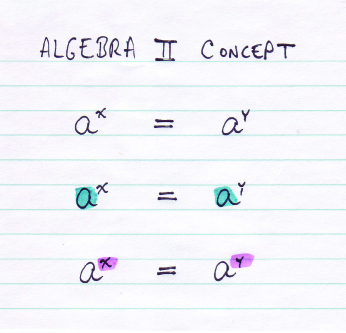The conclusion, of course, is that THEREFORE x = y.

I get a lot of quiet “Now I Get Its” when I show this to Algebra II students.

The “Aha” looks that I get remind me that while this concept is obvious to me and you, many students don’t grasp it fully.

In any case, I wanted to share this because I’ve found that there’s great potential in using color to explain aspects of algebra. I hope that you find this useful, and I encourage you to use color yourself.

Just for the record, the rule I explain in this section is a very useful rule of exponents. To show you its use, I am including the example of a problem that requires its use, below: